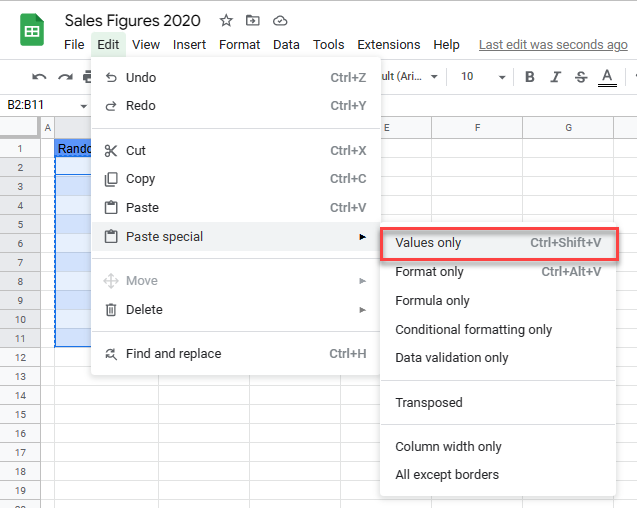# How to Freeze Random Numbers in Excel & Google Sheets

This tutorial demonstrates how to freeze random numbers in Excel and Google Sheets.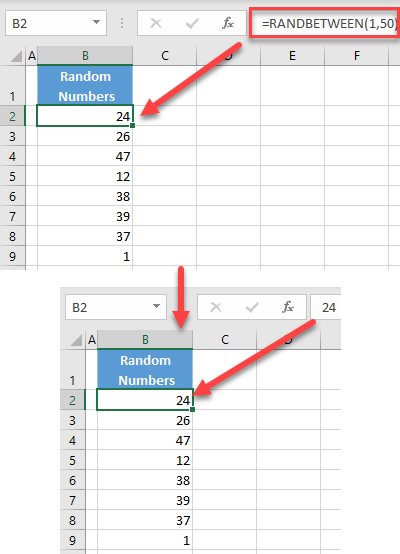If you have a list of random numbers in Excel generated by the RAND Function or RANDBETWEEN Function, every time you edit some value in the workbook, the list automatically updates with new random numbers. To stop this and freeze random numbers, you can copy and paste as values, or set formulas calculation to manual.

## Freeze Random Numbers

### Paste Values

Say you have the following list of random numbers in the range B2:B9, generated with the RANDBETWEEN Function.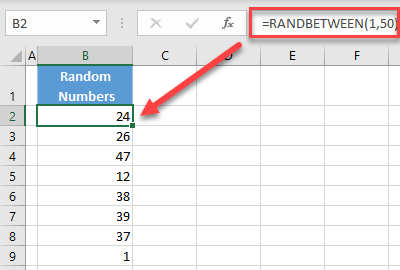If you change any value in the workbook, the list of numbers is regenerated.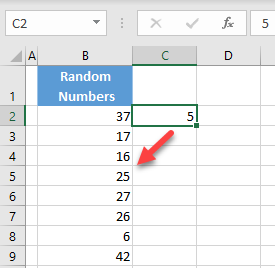To freeze the random numbers, follow these steps:

1. Select the range of cells with random numbers (B2:B9), right-click anywhere in the selected area, and choose Copy (or use the keyboard shortcut CTRL + C).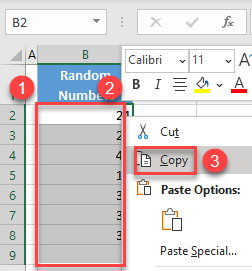1. Right-click the first cell in the range (B2), and choose Values in Paste Options.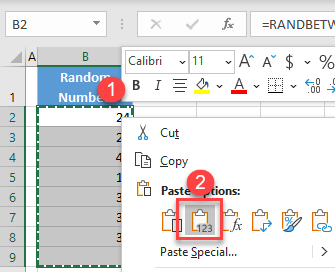As a result, the range is now a list of numbers without a formula. If you change any cell in the worksheet, the values in Column B won’t be changed.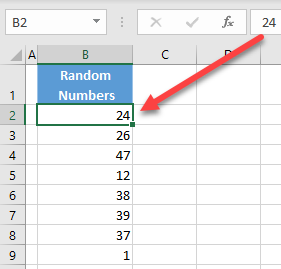### Change Calculation Options

In Excel, formulas calculation is set to automatic, which means that whenever a value is changed in the workbook, all formulas are recalculated. To prevent this, and calculate formulas only when you want, set calculation options to manual. That way, you’ll also freeze your list of random numbers. To achieve this, in the Ribbon, go to Formulas > Calculation Options.Now, although you have the RANDBETWEEN formula in Column B, values are updated only when you click Calculate Now in the Formulas tab or set calculation options back to Automatic.

## Freeze Random Numbers in Google Sheets

In Google Sheets, you can use the Paste values option to freeze random numbers in the way described above, but it’s not possible to set formula calculation to manual.